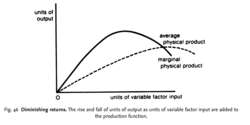# diminishing returns

(redirected from Law of diminishing marginal return)
Also found in: Dictionary.
Related to Law of diminishing marginal return: Marginal product, Law of Diminishing Marginal UtilityFig. 46 Diminishing returns. The rise and fall of units of output as units of variable factor input are added to the production function.

## diminishing returns

the law in the SHORT-RUN theory of supply of diminishing marginal returns or variable factor proportions that states that as equal quantities of one VARIABLE FACTOR INPUT are added into the production function (the quantities of all other factor inputs remaining fixed), a point will be reached beyond which the resulting addition to output (that is, the MARGINAL PHYSICAL PRODUCT of the variable input) will begin to decrease, as shown in Fig. 46.

As the marginal physical product declines, this will eventually cause AVERAGE PHYSICAL PRODUCT to decline as well (diminishing average returns). The marginal physical product changes because additional units of the variable factor input do not add equally readily to units of the fixed factor input.

At a low level of output, marginal physical product rises with the addition of more variable inputs to the (underworked) fixed input, the extra variable inputs bringing about a more intensive use of the fixed input.

Eventually, as output is increased, an optimal factor combination is attained at which the variable and fixed inputs are mixed in the most appropriate proportions to maximize marginal physical product. Thereafter, further additions of variable inputs to the (now overworked) fixed input leads to a less than proportionate increase in output so that marginal physical product declines. See RETURNS TO THE VARIABLE FACTOR INPUT

Collins Dictionary of Economics, 4th ed. © C. Pass, B. Lowes, L. Davies 2005
Site: Follow: Share:
Open / Close